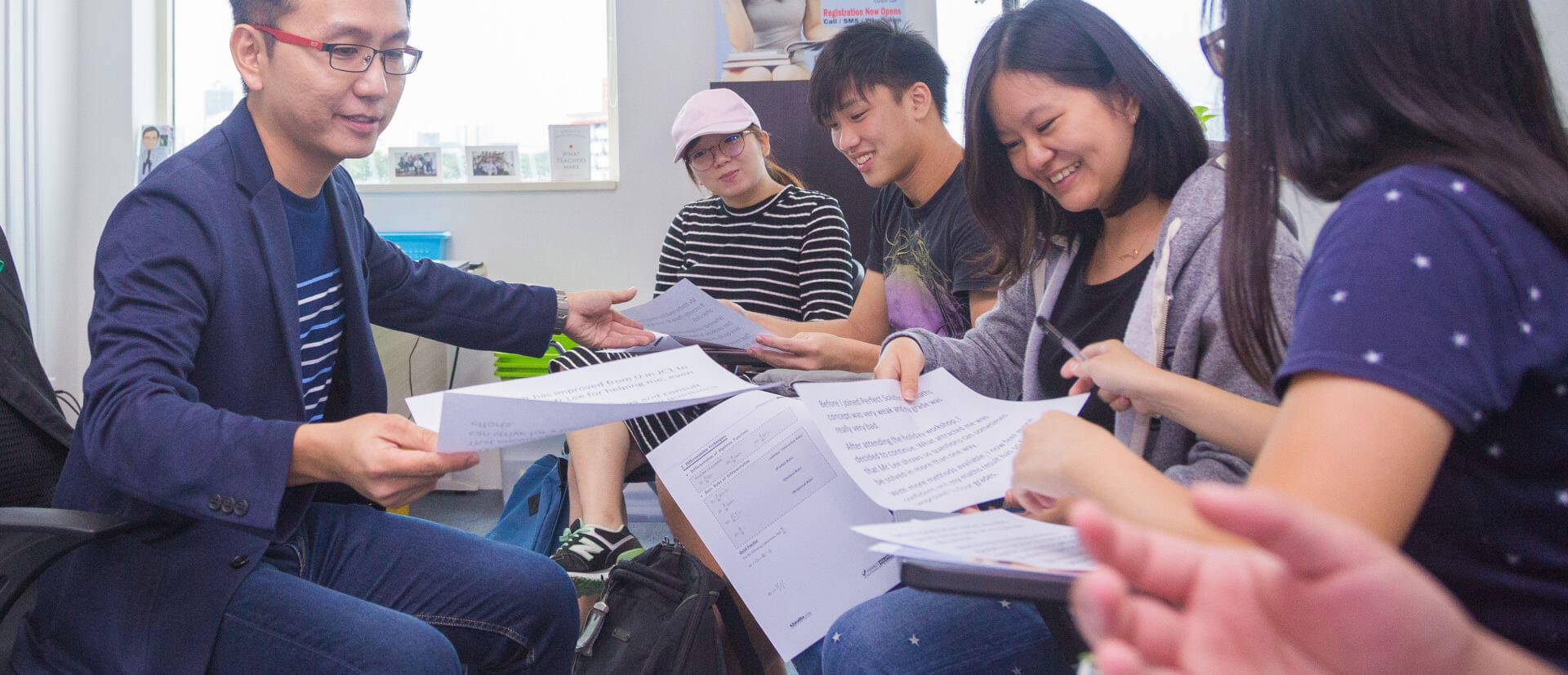# Who were the most famous and important Mathmaticians

Math is an essential science in life as we know it. It is the building blocks to aid in the understanding of the world from tiny atoms to astronomical galaxies. But who were the champions behind the language we call Maths? Here are some of the mathematical heroes that have pushed human progress ahead.

## Euclid

Euclid was one of the most famous mathematicians and he was also known as the “Father of Geometry”! His most famous mathematical contribution is referred to as the Euclidean geometry. Euclidean geometry is the study of plane and solid figures on the basis of axioms and theorems.

## Pythagoras

The pythagoras theorem is popular and taught in most secondary math classes around the world. Pythagoras’ algebraic equations are quite easy and unique, so he was popularly known as the ‘Father of Algebra.’ His most well-known equation is often used in maths calculation from architecture to computer programming.

## Antiphon

Not many may recognise this name but the Greek mathematician Antipon is responsible for discovering the value of Pi. This mathematician was the first one who calculated the upper bound and lowers bound values of Pi by inscribing and circumscribing around a circle, the polygon, and processed finally to calculate the areas of the polygon. His method revolutionlized how we calculate complex shapes.

## Archimedes

Archimedes was possibly the greatest mathematician of all time. He is best known for his contributions in physics by figuring out how levers work and in the famous legend of his discovery of how water is displaced by a submerged object: While taking a bath, Archimedes watched the water sloshing up to the top of his tub, and in the excitement of his discovery, he ran through the streets naked and shouting “Eureka!”

Now you know the most important and famous mathematicians of all time. Understanding these subject pioneers will help understand their mathematical geniuses as well. To think maths, one must think and speak like a mathmatician!

Study up!